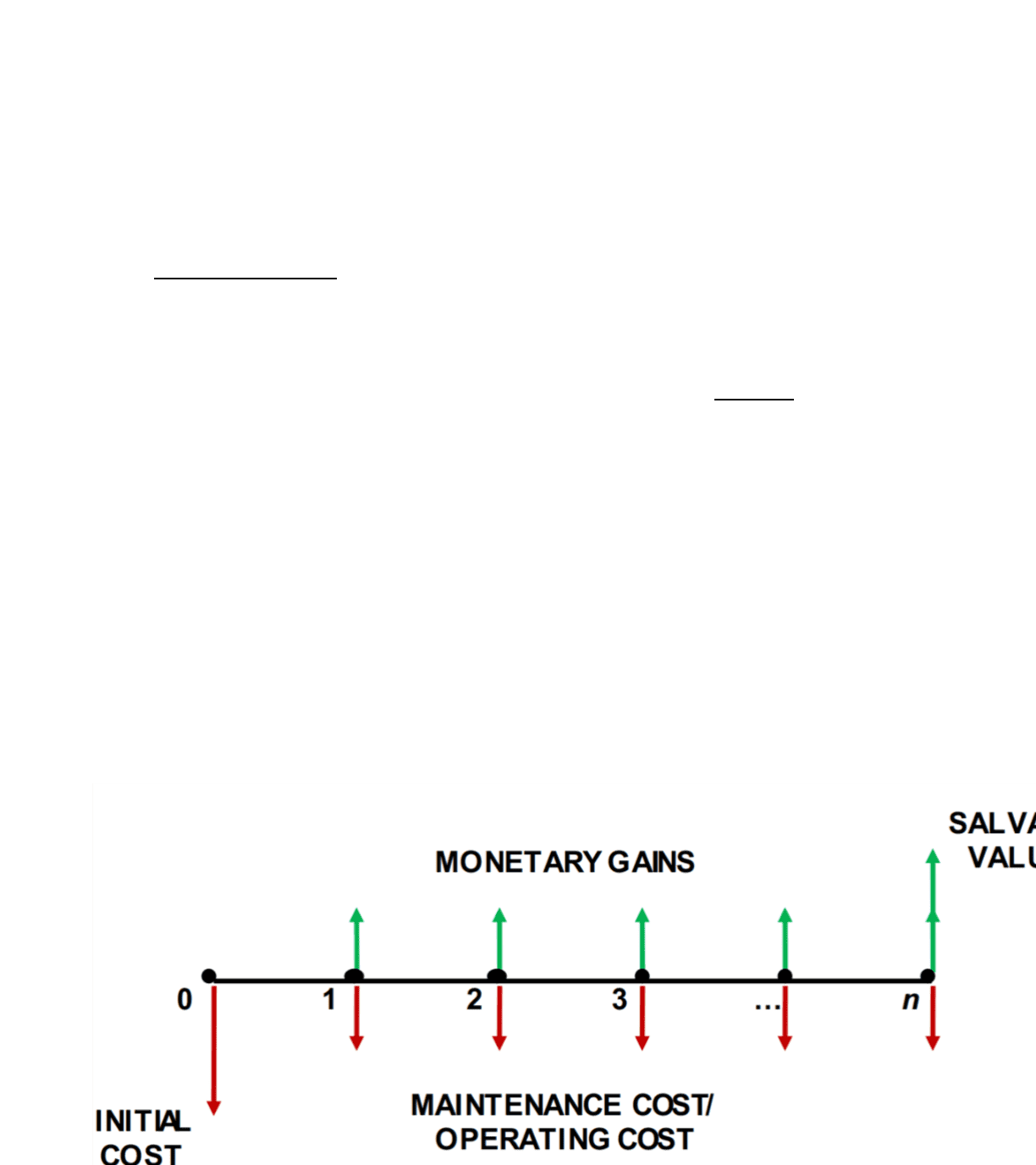Class Notes (1,100,000)
CA (650,000)
Western (60,000)
1220E (100)
Lecture 5

# Business Administration 1220E Lecture Notes - Lecture 5: Engineering Economics, Interest, Cash Flow

Department
Course Code
Professor
Martin Eidenberg
Lecture
5

This preview shows pages 1-2. to view the full 6 pages of the document.Net Present Value & Interest
Net Present Value compares the value of a dollar today to the value of the same
dollar in the future
Takes the difference between the present value of cash inflows and the
present value of cash outflows
Future gains of a dollar amount today are based on the interest earned on that
amount
2 Types of Interest
Simple Interest
Compound Interest
Simple Interest
Interest is paid on the principal but not on any previously earned interest
!"#
\$
%&'(
)
Compound Interest
Interest is earned on the principal and the interest earned in proceeding periods
!"#
\$
%&'
)
*
Cash Flow Diagrams
Net Present Value (NPV)
#" +
!,
\$%&-),
*
,./
An investment is:
Good if NPV > 0
Internal Rate of Return (IRR)
Discount rate at which the NPV of a series of cash flows equals 0
Determines the feasibility of an investment
Good: IRR > MARR
External Rate of Return (ERR)
Rate at which the future value of an investment made at t=0 is equal to the
future value of the reinvested cash flows generated by the investment
Determines the feasibility of an investment
Good: ERR > MARR
Depreciation & Taxes
Depreciation is not a cash flow and should not be included in a NPV analysis
Taxes are real cash flows that need to considered in a NPV analysis
Tax shield: depreciation reduces taxes
We need to adjust for depreciation to determine after-tax cash flow
Public Sector Decisions
The government must evaluate more than just financial considerations
Benefits
Positive factors associated with a project
§
Disbenefits
Negative factors associated with a project
§
Revenues
Actual cash income associated with a project
§
Costs
Actual cash expenses associated with a project
§
The decisions often made are very short-term to be re-elected
Voters tend to be short sighted and generally dislike tax increases for any
reason
Cost-Benefit Analysis
Quantify all benefits and disbenefits in \$ terms
Benefit-Cost Ratio: 012"345\$67879:7;<=<>797?@AB<C<D@;E797?@AB)
345\$FG;A;)
Engineering Economics Math

Only pages 1-2 are available for preview. Some parts have been intentionally blurred.Net Present Value & Interest
Net Present Value compares the value of a dollar today to the value of the same
dollar in the future
Takes the difference between the present value of cash inflows and the
present value of cash outflows
Future gains of a dollar amount today are based on the interest earned on that
amount
2 Types of Interest
Simple Interest
Compound Interest
Simple Interest
Interest is paid on the principal but not on any previously earned interest !"#\$%&'()
Compound Interest
Interest is earned on the principal and the interest earned in proceeding periods !"#\$%&')*
Cash Flow Diagrams
Net Present Value (NPV)
#" +
!,
\$%&-),
*
,./
An investment is:
Good if NPV > 0
Internal Rate of Return (IRR)
Discount rate at which the NPV of a series of cash flows equals 0
Determines the feasibility of an investment
Good: IRR > MARR
External Rate of Return (ERR)
Rate at which the future value of an investment made at t=0 is equal to the
future value of the reinvested cash flows generated by the investment
Determines the feasibility of an investment
Good: ERR > MARR
Depreciation & Taxes
Depreciation is not a cash flow and should not be included in a NPV analysis
Taxes are real cash flows that need to considered in a NPV analysis
Tax shield: depreciation reduces taxes
We need to adjust for depreciation to determine after-tax cash flow
Public Sector Decisions
The government must evaluate more than just financial considerations
Benefits
Positive factors associated with a project
§
Disbenefits
Negative factors associated with a project
§
Revenues
Actual cash income associated with a project
§
Costs
Actual cash expenses associated with a project
§
The decisions often made are very short-term to be re-elected
Voters tend to be short sighted and generally dislike tax increases for any
reason
Cost-Benefit Analysis
Quantify all benefits and disbenefits in \$ terms
Benefit-Cost Ratio: 012"345\$67879:7;<=<>797?@AB<C<D@;E797?@AB)
345\$FG;A;)